As applications engineers, we are constantly bombarded with a variety of questions about driving high-speed analog-to-digital converters (ADCs) with differential inputs. Indeed, selecting the right ADC driver and configuration can be challenging. To make the design of robust ADC circuits somewhat easier, we’ve compiled a set of common “road hazards” and solutions. In this article, the circuit that actually drives the ADC—variously known as an ADC driver, differential amplifier, or diff amp—is assumed to be capable of handling high-speed signals.

### Introduction

Most modern high-performance ADCs use differential inputs to reject common-mode noise and interference, increase dynamic range by a factor of two, and improve overall performance due to balanced signaling. Though ADCs with differential inputs can accept single-ended input signals, optimum ADC performance is achieved when the input signal is differential. ADC drivers—circuits often specifically designed to provide such signals—perform many important functions including amplitude scaling, single-ended-to-differential conversion, buffering, common-mode offset adjustment, and filtering. Since the introduction of the AD8138,1 differential ADC drivers have become essential signal conditioning elements in data-acquisition systems.

A basic fully differential voltage-feedback ADC driver is shown in Figure 1. Two differences from a traditional op-amp feedback circuit can be seen. The differential ADC driver has an additional output terminal (VON) and an additional input terminal (VOCM). These provide great flexibility when interfacing signals to ADCs that have differential inputs.

Instead of a single-ended output, the differential ADC driver produces a balanced differential output—with respect to VOCM—between VOP and VON. “P” indicates positive and “N” indicates negative. The VOCM input controls the output common-mode voltage. As long as the inputs and outputs stay within their specified limits, the output common-mode voltage must equal the voltage applied to the VOCM input. Negative feedback and high open-loop gain cause the voltages at the amplifier input terminals, VA+ and VA–, to be essentially equal.

For the discussions that follow, some definitions are in order. If the input signal is balanced, VIP and VIN are nominally equal in amplitude and opposite in phase with respect to a common reference voltage. When the input is single-ended, one input is at a fixed voltage, and the other varies with respect to it. In either case, the input signal is defined as VIP VIN.

The differential-mode input voltage, VIN, dm, and common-mode input voltage, VIN, cm, are defined in Equation 1 and Equation 2.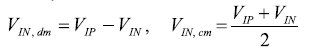(1, 2)

This common-mode definition is intuitive when applied to balanced inputs, but it is also valid for single-ended inputs.

The output also has a differential mode and a common mode, defined in Equation 3 and Equation 4.(3, 4)

Note the difference between the actual output common-mode voltage, VOUT, cm, and the VOCM input terminal, which establishes the output common-mode level.

The analysis of differential ADC drivers is considerably more complex than that of traditional op amps. To simplify the algebra, it is expedient to define two feedback factors, β1 and β2, as given in Equation 5 and Equation 6.(5, 6)

In most ADC driving applications β1 = β2, but the general closed-loop equation for VOUT, dm, in terms of VIP, VIN, VOCM, β1, and β2, is useful to gain insight into how beta mismatch affects performance. The equation for VOUT, dm, shown in Equation 7, includes the finite frequency-dependent open-loop voltage gain of the amplifier, A(s).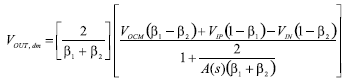(7)

When β1 ≠ β2, the differential output voltage depends on VOCM—an undesirable outcome, since it produces an offset and excess noise in the differential output. The gain-bandwidth product of the voltage-feedback architecture is constant. Interestingly, the gain in the gain-bandwidth product is the reciprocal of the averages of the two feedback factors.

When β1 = β2 ≡ | β, Equation 7 reduces to Equation 8.(8)

This is a more familiar-looking expression; the ideal closed-loop gain becomes simply RF/RG when A(s) → ∞. The gain-bandwidth product is also more familiar-looking, with the “noise gain” equal to 1/β, just as with a traditional op amp.

The ideal closed-loop gain for a differential ADC driver with matched feedback factors is seen in Equation 9.(9)

Output balance, an important performance metric for differential ADC drivers, has two components: amplitude balance and phase balance. Amplitude balance is a measure of how closely the two outputs are matched in amplitude; in an ideal amplifier they are exactly matched. Output phase balance is a measure of how close the phase difference between the two outputs is to 180°. Any imbalance in output amplitude or phase produces an undesirable common-mode component in the output. The output balance error (Equation 10) is the log ratio of the output common-mode voltage produced by a differential input signal to the output differential-mode voltage produced by the same input signal, expressed in dB.(10)

An internal common-mode feedback loop forces VOUT, cm to equal the voltage applied to the VOCM input, producing excellent output balance.

### Terminating the Input to an ADC Driver

ADC drivers are frequently used in systems that process high-speed signals. Devices separated by more than a small fraction of a signal wavelength must be connected by electrical transmission lines with controlled impedance to avoid losing signal integrity. Optimum performance is achieved when a transmission line is terminated at both ends in its characteristic impedance. The driver is generally placed close to the ADC, so controlled-impedance connections are not required between them; but the incoming signal connection to the ADC driver input is often long enough to require a controlled-impedance connection, terminated in the proper resistance.

The input resistance of the ADC driver, whether differential or single-ended, must be greater than or equal to the desired termination resistance, so that a termination resistor, RT, can be added in parallel with the amplifier input to achieve the required resistance. All ADC drivers in the examples considered here are designed to have balanced feedback ratios, as shown in Figure 2.

Because the voltage between the two amplifier inputs is driven to a null by negative feedback, they are virtually connected, and the differential input resistance, RIN, is simply 2 × RG. To match the transmission-line resistance, RL, place resistor, RT, as calculated in Equation 11, across the differential input. Figure 3 shows typical resistances RF = RG = 200 Ω, desired RL, dm = 100 Ω, and RT = 133 Ω.(11)

Terminating a single-ended input requires significantly more effort. Figure 4 illustrates how an ADC driver operates with a single-ended input and a differential output.

Although the input is single-ended, VIN, dm is equal to VIN. Because resistors RF and RG are equal and balanced, the gain is unity, and the differential output, VOP – VON, is equal to the input, that is, 4 V p-p. VOUT, cm is equal to VOCM = 2.5 V and, from the lower feedback circuit, input voltages VA+ and VA– are equal to VOP/2.

Using Equation 3 and Equation 4, VOP = VOCM + VIN/2, an in-phase swing of ±1 V about 2.5 V. VON = VOCM – VIN/2, an antiphase-swing of ±1 V about 2.5 V. Thus, VA+ and VA– swing ±0.5 V about 1.25 V. The ac component of the current that must be supplied by VIN is (2 V – 0.5 V)/500 Ω = 3 mA, so the resistance to ground that must be matched, looking in from VIN, is 667 Ω.

The general formula for determining this single-ended input resistance when the feedback factors of each loop are matched is shown in Equation 12, where RIN, se is the single-ended input resistance.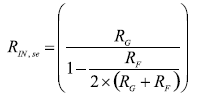(12)

This is a starting point for calculating the termination resistance. However, it is important to note that amplifier gain equations are based on the assumption of a zero-impedance input source. A significant source impedance that must be matched in the presence of an imbalance caused by a single-ended input inherently adds resistance only to the upper RG. To retain the balance, this must be matched by adding resistance to the lower RG, but this affects the gain.

While it may be possible to determine a closed-form solution to the problem of terminating a single-ended signal, an iterative method is generally used. The need for it will become apparent in the following example.

In Figure 5, a single-ended-to-differential gain of one, a 50 Ω input termination, and feedback and gain resistors with values in the neighborhood of 200 Ω are required to keep noise low.

Equation 12 provides the single-ended input resistance, 267 Ω. Equation 13 indicates that the parallel resistance, RT, should be 61.5 Ω to bring the 267-Ω input resistance down to 50 Ω.(13)

Figure 6 shows the circuit with source and termination resistances. The open-circuit voltage of the source, with its 50-Ω source resistance, is 2 V p-p. When the source is terminated in 50 Ω, the input voltage is reduced to 1 V p-p, which is also the differential output voltage of the unity-gain driver.

This circuit may initially appear to be complete, but an unmatched resistance of 61.5 Ω in parallel with 50 Ω has been added to the upper RG alone. This changes the gain and single-ended input resistance, and mismatches the feedback factors. For small gains, the change in input resistance is small and will be neglected for the moment, but the feedback factors must still be matched. The simplest way to accomplish this is to add resistance to the lower RG. Figure 7 shows a Thévenin equivalent circuit in which the above parallel combination acts as the source resistance.

With this substitution, a 27.6-Ω resistor, RTS, is added to the lower loop to match loop feedback factors, as seen in Figure 8.

Note that the Thévenin voltage of 1.1 V p-p is larger than the properly terminated voltage of 1 V p-p, while the gain resistors are each increased by 27.6 Ω, decreasing the closed-loop gain. These opposing effects tend to cancel for large resistors (>1 kΩ) and small gains (1 or 2), but do not entirely cancel for small resistors or higher gains.

The circuit in Figure 8 is now easily analyzed, and the differential output voltage is calculated in Equation 14.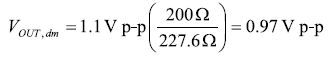(14)

The differential output voltage is not quite at the desired level of 1 V p-p, but a final independent gain adjustment is available by modifying the feedback resistance as shown in Equation 15.(15)

Figure 9 shows the completed circuit, implemented with standard 1% resistor values.

Observations: Referring to Figure 9, the single-ended input resistance of the driver, RIN, se, has changed due to changes in RF and RG. The driver’s gain resistances are 200 Ω in the upper loop and 200 Ω + 28 Ω = 228 Ω in the lower loop. Calculation of RIN, se with differing gain resistance values first requires two values of beta to be calculated, as shown in Equation 16 and Equation 17.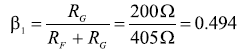(16)(17)

The input resistance, RIN, se, is calculated as shown in Equation 18.(18)

This differs little from the original calculated value of 267 Ω, and does not have a significant effect on the calculation of RT, since RIN, se is in parallel with RT.

If a more-exact overall gain were necessary, higher precision or series trim resistors could be used.

A single iteration of the method described here works well for closed-loop gains of one or two. For higher gains, the value of RTS gets closer to the value of RG, and the difference between the value of RIN, se calculated in Equation 18 and that calculated in Equation 12 becomes greater. Several iterations are required for these cases.

This should not be arduous: Recently released differential amplifier calculator tools, ADIsimDiffAmp™ (Ref. 2) and ADI Diff Amp Calculator™(Ref. 3) downloadable, do all the heavy lifting; they will perform the above calculations in a matter of seconds.

### Input Common-Mode Voltage Range

Input common-mode voltage range (ICMVR) specifies the range of voltage that can be applied to the differential-amplifier inputs for normal operation. The voltage appearing at those inputs can be referred to as ICMV, Vacm, or V. This specification is often misunderstood. The most frequent difficulty is determining the actual voltage at the differential amplifier inputs, especially with respect to the input voltage. The amplifier input voltage (V) can be calculated knowing the variables VIN, cm, β, and VOCM, using the general Equation 19 for unequal β’s, or the simplified Equation 20 for equal β’s.(19)(20)

It may be useful to recall that VA is always a scaled-down version of the input signal (as seen in Figure 4). The input common-mode voltage range differs among amplifier types. Analog Devices high-speed differential ADC drivers have two input stage configurations, centered and shifted. The centered ADC drivers have about 1 V of headroom from each supply rail (hence centered). The shifted input stages add two transistors to allow the inputs to swing closer to the –VS rail. Figure 10 shows a simplified input schematic of a typical differential amplifier (Q2 and Q3).

The shifted input architecture allows the differential amplifier to process a bipolar input signal, even when the amplifier is powered from a single supply, making them well suited for single-supply applications with inputs at or below ground. The additional PNP transistor (Q1 and Q4) at the input shifts the input to the differential pair up by one transistor Vbe. For example, with –0.3 V applied at –IN, point A would be 0.7 V, allowing the differential pair to operate properly. Without the PNPs (centered input stage), –0.3 V at point A would reverse bias the NPN differential pair and halt normal operation.

Table 1 provides a quick reference to many specifications of Analog Devices ADC drivers. A glance reveals the drivers that feature a shifted ICMVR and those that do not.

Table 1. High-Speed ADC Driver Specifications

### Input and Output Coupling: AC or DC

The need for ac or dc coupling can have a significant impact on the choice of a differential ADC driver. The considerations differ between input and output coupling.

An ac-coupled input stage is illustrated in Figure 11.

For differential-to-differential applications with ac-coupled inputs, the dc common-mode voltage appearing at the amplifier input terminals is equal to the dc output common-mode voltage, since dc feedback current is blocked by the input capacitors. Also the feedback factors at dc are matched and exactly equal to unity. VOCM—and consequently the dc input common-mode—is very often set near midsupply. An ADC driver with centered input common-mode range works well in these types of applications, with the input common-mode voltage near the center of its specified range.

AC-coupled single-ended-to-differential applications are similar to their differential-input counterparts but have common-mode ripple—a scaled-down replica of the input signal—at the amplifier input terminals. An ADC driver with centered input common-mode range places the average input common-mode voltage near the middle of its specified range, providing plenty of margin for the ripple in most applications.

When input coupling is optional, it is worth noting that ADC drivers with ac-coupled inputs dissipate less power than similar drivers with dc-coupled inputs, since no dc common-mode current flows in either feedback loop.

AC coupling the ADC driver outputs is useful when the ADC requires an input common-mode voltage that differs substantially from that available at the output of the driver. The drivers have maximum output swing when VOCM is set near midsupply; this presents a problem when driving low-voltage ADCs with very low input common-mode voltage requirements. A simple solution to this predicament (Figure 12) is to ac-couple the connection between the driver output and the ADC input, removing the ADC’s dc common-mode voltage from the driver output, and allowing a common-mode level suitable for the ADC to be applied on its side of the ac-coupling. For example, the driver could be running on a single 5-V supply with VOCM = 2.5 V, and the ADC could be running on a single 1.8-V supply with a required input common-mode voltage of 0.9 V applied at the point labeled ADC CMV.

Drivers with shifted input common-mode ranges generally work best in dc-coupled systems operating on single supplies. This is because the output common-mode voltage gets divided down through the feedback loops, and its variable components can get close to ground, which is the negative rail. With single-ended inputs, the input common-mode voltage gets even closer to the negative rail due to the input-related ripple.

Systems running on dual supplies, with single-ended or differential inputs and ac- or dc-coupling, are usually fine with either type of input stage because of the increased headroom.

Table 2 summarizes the most common ADC driver input-stage types used with various input-coupling and power-supply combinations. However, these choices may not always be the best; each system should be analyzed on a case-by-case basis.

Table 2. Coupling and Input-Stage Options

 Input Coupling Input Signal Power Supplies Input Type Any Any Dual Either Either AC Single-Ended Single Centered DC Single-Ended Single Shifted AC Differential Single Centered DC Differential Single Centered

### Output Swing

To maximize the dynamic range of an ADC, it should be driven to its full input range. But care is needed: drive the ADC too hard and risk damaging the input, not hard enough and resolution is lost. Driving the ADC to its full input range does not mean that the amplifier output has to swing to its full range. A major benefit of differential outputs is that each output only has to swing half as much as a traditional single-ended output. The driver outputs can stay away from the supply rails, allowing decreased distortion. This is not the case for single-ended drivers, however. As the output voltage of the driver approaches the rail, the amplifier loses linearity and introduces distortion.

For applications where every last millivolt of output voltage is required, Table 1 shows that quite a few ADC drivers have rail-to-rail outputs, with typical headroom ranging from a few millivolts to a few hundred millivolts, depending on the load.

Figure 13 shows a plot of harmonic distortion vs. VOCM at various frequencies for the ADA4932, which is specified with a typical output swing to within 1.2 V of each rail (headroom). The output swing is the sum of VOCM and VPEAK of the signal (1 V). Note that the distortion starts to take off above 2.8 V (3.8-VPEAK, or 1.2 V below the 5-V rail). At the low end, distortion is still low at 2.2 V (–1 VPEAK). The same type of behavior will appear in the discussions of bandwidth and slew rate.

### Noise

ADC imperfections include quantization noise, electronic—or random—noise, and harmonic distortion. Important in most applications, noise is usually the most important performance metric in broadband systems.

All ADCs inherently have quantization noise, which depends on the number of bits, n, decreasing with increasing n. Because even “ideal” converters produce quantization noise, it will be used as a benchmark against which to compare random noise and harmonic distortion. The output noise from the ADC driver should be comparable to or lower than the ADC’s random noise and distortion. Beginning with a review of the characterization of ADC noise and distortion, we will then show how to weigh ADC driver noise against the ADC’s performance.

Quantization noise occurs because the ADC quantizes analog signals having infinite resolution into a finite number of discrete levels. An n-bit ADC has 2n binary levels. The difference between one level and the next represents the finest difference that can be resolved; it is referred to as a least significant bit (LSB), or q, for quantum level. One quantum level is therefore 1/2n of the converter’s range. If a varying voltage is converted by a perfect n-bit ADC, then converted back to analog and subtracted from the ADC’s input, the difference will look like noise. It will have an rms value of (Equation 21):(21)

From this, the logarithmic (dB) formula for the signal-to-quantizing-noise ratio of an n-bit ADC over its Nyquist bandwidth can be derived (Equation 22); it is the best achievable SNR for an n-bit converter.

Random noise in ADCs, a combination of thermal, shot, and flicker noise, is generally larger than the quantization noise. Harmonic distortion, resulting from nonlinearities in the ADC, produces unwanted signals in the output that are harmonically related to the input signals. Total harmonic distortion and noise (THD + N) is an important ADC performance metric that compares the electronic noise and harmonic distortion to an analog input that is close to the full-scale input range of the ADC. Electronic noise is integrated over a bandwidth that includes the frequency of the last harmonic to be considered. Here, the “total” in THD includes the first five harmonic-distortion components, which are root-sum-squared along with the noise (Equation 23).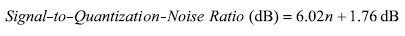(22)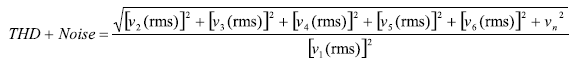(23)

The input signal is v1; the first five harmonic-distortion products are v2 through v6; and the ADC electronic noise is vn.

The reciprocal of THD + Noise, the signal-to-noise-and-distortion ratio, or SINAD, is usually expressed in dB (Equation 24).(24)

If SINAD is substituted for the signal-to-quantizing-noise ratio (Equation 22), we can define an effective number of bits (ENOB) that a converter would have if its signal-to-quantizing-noise ratio were the same as its SINAD (Equation 25).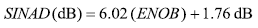(25)

ENOB can also be expressed in terms of SINAD as shown in Equation 26.(26)

ENOB can be used to compare noise performance of an ADC driver with that of the ADC to determine its suitability to drive that ADC. A differential ADC noise model is shown in Figure 14.

The contributions to the total output noise density of each of the eight sources are shown in Equation 27 for the general case, and when β1 = β2 ≡ β.(27)

The total output noise voltage density, vno, dm, is calculated by computing the root sum square of these components. Entering the equations into a spreadsheet is the best way to calculate the total output noise voltage density. The new ADI Diff Amp Calculator (Ref. 3), which will quickly calculate noise, gain, and other differential ADC driver behavior, is also available on the Analog Devices website.

ADC driver noise performance can now be compared with the ENOB of an ADC. An example that illustrates this procedure is to select and evaluate a differential driver with a gain of 2 for an AD9445 ADC on a 5-V supply, with a 2-V full-scale input; it is processing a direct-coupled broadband signal occupying a 50 MHz (–3-dB) bandwidth, limited with a single-pole filter. From the data-sheet listing of ENOB specifications for various conditions: for a Nyquist bandwidth of 50 MHz, ENOB = 12 bits.

The ADA4939 is a high-performance broadband differential ADC driver that can be direct-coupled. Is it a good candidate to drive the AD9445 with respect to noise? The data sheet recommends RF = 402 Ω and RG = 200 Ω, for a differential gain of approximately 2. The data sheet gives a total output voltage noise density for this case as 9.7 nV√Hz.

First, calculate the system noise bandwidth, BN, which is the bandwidth of an equivalent rectangular low-pass filter that outputs the same noise power as the actual filter that determines the system bandwidth, for a given constant input noise power spectral density. For a one-pole filter, BN is equal to π/2 times the 3-dB bandwidth, as shown here (Equation 28).(28)

Next, integrate the noise density over the square-root of the system bandwidth to obtain the output rms noise (Equation 29).(29)

The amplitude of the noise is presumed to have a Gaussian distribution, so, using the common ±3σ limits for the peak-to-peak noise (noise voltage swings between these limits about 99.7% of the time), the peak-to-peak output noise is calculated in Equation 30.(30)

Now compare the driver’s peak-to-peak output noise with 1 LSB voltage of the AD9445 LSB, based on an ENOB of 12 bits and full-scale input range of 2 V, as calculated in Equation 31.(31)

The peak-to-peak output noise from the driver is comparable to the ADC’s LSB, with respect to 12 bits of ENOB; the driver is therefore a good choice to consider in this application from the standpoint of noise. The final determination must be made by building and testing the driver/ADC combination.

### Supply Voltage

Considering supply voltage and current is a quick way to narrow the choice of ADC drivers. Table 1 provides a compact reference to ADC driver performance with respect to power supply. The supply voltage influences bandwidth and signal swing, as well as ICMVR. Weighing the specifications and reviewing the trade-offs are important to diff amp selection.

Power-supply rejection (PSR) is another important specification. The role of power-supply pins as inputs to the amplifier is often ignored. Any noise on the power-supply lines or coupled into them can potentially corrupt the output signal.

For example, consider the ADA4937-1 with 50 mV p-p at 60 MHz of noise on the power line. Its PSR at 50 MHz is –70 dB. This means the noise on the power supply line would be reduced to approximately 16 μV at the amplifier output. In a 16-bit system with a 1-V full-scale input, 1 LSB is 15.3 μV; the noise from the power supply line would therefore swamp the LSB.

This situation can be improved by adding series SMT ferrite beads, L1/L2, and shunt bypass capacitors, C1/C2 (Figure 15).

At 50 MHz, the ferrite bead has an impedance of 60 Ω and the 10-nF (0.01-μF) capacitor has an impedance of 0.32 Ω. The attenuator formed by these two elements provides 45.5 dB of attenuation (Equation 32).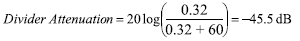(32)

The divider attenuation combines with the PSR of –70 dB to provide about 115 dB of rejection. This reduces the noise to approximately 90 nV p-p, well below 1 LSB.

### Harmonic Distortion

Low harmonic distortion in the frequency domain is important in both narrow-band and broadband systems. Nonlinearities in the drivers generate single-tone harmonic distortion and multitone intermodulation-distortion products at amplifier outputs.

The same approach used in the noise-analysis example can be applied to distortion analysis, comparing the ADA4939’s harmonic distortion with 1 LSB of the AD9445’s ENOB of 12 bits with 2-V full-scale output. One ENOB LSB was shown to be 488 μV in the noise analysis.

The distortion data in the ADA4939 specification table is given for a gain of 2, comparing 2nd and 3rd harmonics at various frequencies. Table 3 shows the harmonic distortion data for a gain of 2 and differential output swing of 2 V p-p.

Table 3. ADA4939 Second and Third Harmonic Distortion

 Parameter Harmonic Distortion HD2 @ 10 MHz –102 dBc HD2 @ 70 MHz –83 dBc HD2 @ 70 MHz –83 dBc HD2 @ 100 MHz –77 dBc HD2 @ 10 MHz –101 dBc HD2 @ 70 MHz –97 dBc HD2 @ 100 MHz –97 dBc

The data show that harmonic distortion increases with frequency and that HD2 is worse than HD3 in the bandwidth of interest (50 MHz). Harmonic distortion products are higher in frequency than the frequency of interest, so their amplitude may be reduced by system band-limiting. If the system had a brick-wall filter at 50 MHz, only the frequencies higher than 25 MHz would be of concern, since all harmonics of higher frequencies would be eliminated by the filter. Nevertheless, we will evaluate the system up to 50 MHz, since any filtering that is present may not sufficiently suppress the harmonics, and distortion products can alias back into the signal bandwidth. Figure 16 shows the ADA4939’s harmonic distortion vs. frequency for various supply voltages with a 2 V p-p output.

HD2 at 50 MHz is approximately –88 dBc, relative to a 2-V p-p input signal. In order to compare the harmonic distortion level to 1 ENOB LSB, this level must be converted to a voltage as shown in Equation 33.(33)

This distortion product is only 80 μV p-p, or 16% of 1 ENOB LSB. Thus, from a distortion standpoint, the ADA4939 is a good choice to consider as a driver for the AD9445 ADC.

Since ADC drivers are negative feedback amplifiers, output distortion depends upon the amount of loop gain in the amplifier circuit. The inherent open-loop distortion of a negative feedback amplifier is reduced by a factor of 1/(1 + LG), where LG is the available loop gain.

The amplifier’s input (error voltage) is multiplied by a large forward voltage gain, A(s), then passes though the feedback factor, β, to the input, where it adjusts the output to minimize the error. Thus, the loop gain of this type of amplifier is A(s) × β; as the loop gain (A(s), β, or both) decreases, harmonic distortion increases. Voltage-feedback amplifiers, like integrators, are designed to have large A(s) at dc and low frequencies, then roll off as 1/f toward unity at a specified high frequency. As A(s) rolls off, loop gain decreases and distortion increases. Thus, the harmonic distortion characteristic is the inverse of A(s).

Current-feedback amplifiers use an error current as the feedback signal. The error current is multiplied by a large forward transresistance, T(s), which converts it to the output voltage, then passes through the feedback factor, 1/RF, which converts the output voltage to a feedback current that tends to minimize the input error current. The loop gain of an ideal current feedback amplifier is therefore T(s) × (1/RF) = T(s)/RF. Like A(s), T(s) has a large dc value and rolls off with increasing frequency, reducing loop gain and increasing the harmonic distortion.

Loop gain also depends directly upon the feedback factor, 1/RF. The loop gain of an ideal current-feedback amplifier does not depend upon a closed-loop voltage gain, so harmonic distortion performance does not degrade as closed-loop gain is increased. In a real current-feedback amplifier, loop gain does have some dependence on closed-loop gain, but not nearly to the extent that it does in a voltage-feedback amplifier. This makes a current-feedback amplifier, such as the ADA4927, a better choice than a voltage-feedback amplifier for applications requiring high closed-loop gain and low distortion. Figure 17 shows how well distortion performance holds up as closed-loop gain is increased.

### Bandwidth and Slew Rate

Bandwidth and slew rate are especially important in ADC driver applications. Typically, bandwidth of a device is used to mean the small-signal bandwidth, while slew rate measures the maximum rate of change at the amplifier output for large signal swings.

Effective usable bandwidth (EUBW), a new acronym analogous to ENOB (effective number of bits), describes bandwidth. Many ADC drivers and op amps boast wide bandwidth specs, but not all that bandwidth is usable. For example,–3-dB bandwidth is a conventional way to measure bandwidth, but it doesn’t mean that all the bandwidth is usable. The –3-dB bandwidth’s amplitude and phase errors can be seen a decade earlier than the actual “break” frequency. So what is the EUBW of an amplifier and how is it determined? An excellent way to determine the usable bandwidth is to consult the distortion plots on the data sheet.

Figure 18 indicates that in order to maintain greater than –80 dBc for 2nd and 3rd harmonics, this ADC driver shouldn’t be used for frequencies greater than 60 MHz. Since each application is different, the system requirements will be a guide to the appropriate driver with sufficient bandwidth and adequate distortion performance.

Slew rate, a large signal parameter, refers to the max rate of change the amplifier output can track the input without excessive distortion. Consider the sine wave output at the slew rate.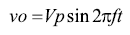(34)

The derivative (rate of change) of Equation 34 at the zero crossing, the maximum rate, is(35)

Where dv/dt max is the slew rate, Vp is the peak voltage, and f equals the full-power bandwidth (FPBW). Solving for FPBW,(36)

Therefore, when selecting an ADC driver, it is important to consider the gain, bandwidth, and slew rate (FPBW) to determine if the amplifier is adequate for the application.

### Stability

Stability considerations for differential ADC drivers are the same as for op amps. The key specification is phase margin. While the phase margin of a particular amplifier configuration can be determined from the data sheets, in a real system it can be significantly reduced by parasitic effects in the PC board layout.

Stability of a negative-voltage-feedback amplifier depends on the magnitude and sign of its loop gain, A(s) × β. The differential ADC driver is a bit more complicated than a typical op-amp circuit, because it has two feedback factors. Loop gain is seen in the denominators of Equation 7 and Equation 8. Equation 37 describes the loop gain for the unmatched feedback factor case (β1 ≠ β2).(37)

With unmatched feedback factors, the effective feedback factor is simply the average of the two feedback factors. When they are matched and defined as β, the loop gain simplifies to A(s) × β.

For a feedback amplifier to be stable, its loop gain must not be allowed to equal –1; or its equivalent, an amplitude of 1 with phase shift of –180°. For a voltage feedback amplifier, the point where the magnitude of loop gain equals 1 (that is, 0 dB) on its open-loop gain-frequency plot is where the magnitude of A(s) equals the reciprocal of the feedback factor. For basic amplifier applications, the feedback is purely resistive, introducing no phase shifts around the feedback loop. With matched feedback factors, the frequency independent reciprocal of the feedback factor, 1 + RF/RG, is often referred to as the noise gain. If the constant noise gain in dB is plotted on the same graph as the open-loop gain, A(s), the frequency where the two curves intersect is where the loop gain is 1, or 0 dB. The difference between the phase of A(s) at that frequency and –180°; is defined as the phase margin; for stable operation, it should be greater than or equal to 45°. Figure 19 illustrates the unity-loop-gain point and phase margin for the ADA4932 with RF/RG = 1 (noise gain = 2).

Further examination of Figure 19 shows that the ADA4932 has approximately 50° of phase margin at a noise gain of 1 (100% feedback in each loop). While it is not practical to operate the ADC drivers at zero gain, this observation shows that the ADA4932 can operate stably at fractional differential gains (RF/RG = 0.25, noise gain = 1.25, for example). This is not true for all differential ADC drivers. Minimum stable gains can be seen in all ADC driver data sheets.

Phase margin for current-feedback ADC drivers can also be determined from open-loop responses. Instead of forward gain, A(s), current-feedback amplifiers use forward transimpedance, T(s), with an error current as the feedback signal. The loop gain of a current-feedback driver with matched feedback resistors is T(s)/RF, so the magnitude of the current-feedback amplifier loop gain is equal to 1 (that is, 0 dB) when T(s) = RF. This point can be easily located on the open-loop transimpedance and phase plot, in the same way as for the voltage-feedback amplifier. Note that plotting the ratio of a resistance to 1 kΩ allows resistances to be expressed on a log plot. Figure 20 illustrates the unity loop-gain point and phase margin of the ADA4927 current-feedback differential ADC driver with RF = 300 Ω.

The loop gain is 0 dB where the 300 Ω feedback resistance horizontal line intersects the transimpedance magnitude curve. At this frequency, the phase of T(s) is approximately –135°, resulting in phase margin of 45°. Phase margin and stability increase as RF increases, and decrease as RF decreases. Current-feedback amplifiers should always use purely resistive feedback with sufficient phase margin.

### PCB Layout

Once a stable ADC driver is designed, it must be realized on a PC board. Some phase margin will always be lost because of the board’s parasitic elements, which must be kept to a minimum. Of particular concern are load capacitance, feedback loop inductance, and summing-node capacitance. Each of these parasitic reactances adds lagging phase shift to the feedback loops, thereby reducing phase margin. A design may lose 20° or more of phase margin due to poor PC board layout.

With voltage-feedback amplifiers, it is best to use the smallest possible RF in order to minimize the phase shift due to the pole formed by RF and the summing-node capacitance. If large RF is required, that capacitance can compensated with small capacitors, CF, across each feedback resistor with values such that RFCF equals RG times the summing node capacitance.

PCB layout is necessarily one of the last steps in a design. Unfortunately, it is also one of the most often overlooked steps in a design, even though high-speed circuit performance is highly dependent on layout. A high-performance design can be compromised, or even rendered useless, by a sloppy or poor layout. Although all aspects of proper high-speed PCB design can’t be covered here, a few key topics will be addressed.

Parasitic elements rob high-speed circuits of performance. Parasitic capacitance is formed by component pads and traces and ground or power planes. Long traces without ground plane will form parasitic inductances, which can lead to ringing in transient responses and other unstable behavior. Parasitic capacitance is especially dangerous at the summing nodes of an amplifier, by introducing a pole in the feedback response, causing peaking and instability. One solution is to make sure that the areas beneath the ADC driver mounting and feedback component pads are cleared of ground and power planes throughout all layers of the board.

Minimizing undesired parasitic reactances starts with keeping all traces as short as possible. Outer layer 50-Ω PC-board traces on FR-4 contribute roughly 2.8 pF/inch and 7 nH/inch. These parasitic reactances increase by about 30% for inner-layer 50 Ω traces. Also make sure there is ground plane under long traces to minimize trace inductance. Keeping traces short and small will help minimize both parasitic capacitance and inductance—and maintain the design’s integrity.

Power-supply bypassing is another key area of concern for layout; make sure the power supply bypass capacitors, as well as the VOCM bypass capacitor, are located as close to the amplifier pins as possible. Also, using multiple bypass capacitors on the power supplies will help ensure that a low impedance path is provided for broadband noise. Figure 21 shows a typical diff amp schematic with bypassing and output low-pass filters. The low-pass filter, limits the bandwidth and noise entering the ADC. Ideally, the power supply bypass-capacitor returns are close to the load returns; this helps reduce circulating currents in the ground plane and improves ADC driver performance. (Figure 22a and Figure 22b).

Use of ground plane, and grounding in general, is a detailed and complex subject and beyond the scope of this article. However, there are a few key points to make, which are illustrated in Figure 22a and Figure 22b. First, connect the analog and digital grounds together at only one point and one point only. This will minimize the interaction of analog and digital currents flowing in the ground plane, which would ultimately lead to “noise” in the system. Also, terminate the analog power supply into the analog power plane and the digital power supply into the digital power plane. For mixed-signal ICs, terminate the analog returns in the analog ground plane and the digital ground return in the digital ground plane.